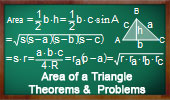# Plane Geometry: Area of a triangle, Theorems and Problems - Table of Content - Page 5Proposed Problem 161. Parallelogram, Midpoints, Area of an Octagon, Area of triangles.

Proposed Problem 147. Quadrilateral, Area, Midpoints, Triangle.

Proposed Problem 146. Varignon's Theorem: Quadrilateral, Midpoints, Parallelogram, Area, Perimeter. Triangles.

Proposed Problem 142. Four Triangles, Incircle, Tangent and Parallel to Side, Areas.

Proposed Problem 139. Triangle Area, Orthic Triangle, Semiperimeter, Circumradius.

Proposed Problem 125. Area of Triangle, Star, Trisection of Sides.

Proposed Problem 124. Area of triangle, Similarity, Trisection of Sides.

Proposed Problem 123. Area of triangle, Similarity, Trisection of Sides.

Proposed Problem 122. Marion Walter's Theorem. Area of triangle and Hexagon, Trisection of Sides.

Go to Page: Previous | 1 | 2 | 3 | 4 | 5 | 6 | 7 | 8 | Next